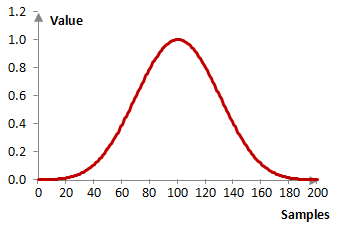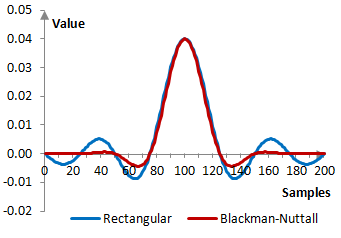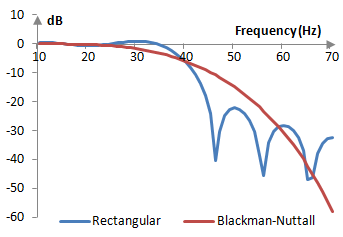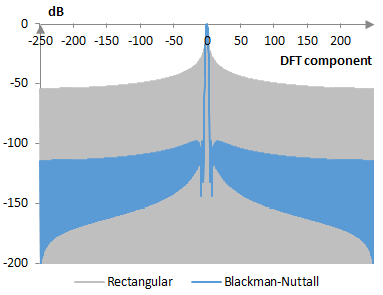# Blackman-Nuttall window

The Blackman-Nuttall window coefficients are given by the following formula

$$a(k)=0.3635819-0.4891775 \, \cos(\frac{2\pi k}{N-1})$$ $$+0.1365995 \, \cos(\frac{4\pi k}{N-1})-0.0106411 \, \cos(\frac{6\pi k}{N-1})$$

where N is the length of the filter and k = 0, 1, …, N – 1.

The Blackman-Nuttall window is a generalized cosine window (see Hamming window).

## An example Blackman-Nuttall window

Consider a finite impulse response (FIR) low pass filter of length N = 201. The following is the Blackman-Nuttall window.Given a sampling frequency of 2000 Hz and a filter cutoff frequency of 40 Hz, the impulse response of the filter with a rectangular window (with no window) and with the Blackman-Nuttall window is as follows.The magnitude response of the same filter is shown on the graph below.## Measures for the Blackman-Nuttall window

The following graph compares the discrete Fourier transform of the Blackman-Nuttall window and the rectangular window.The Blackman-Nuttall window measures are as follows.

 Coherent gain 0.36 Equivalent noise bandwidth 1.98 Processing gain -2.97 dB Scalloping loss -0.85 dB Worst case processing loss -3.81 dB Highest sidelobe level -98.3 dB Sidelobe falloff -12.7 dB / octave, -42.2 dB / decade Main lobe is -3 dB 1.88 bins Main lobe is -6 dB 2.62 bins Overlap correlation at 50% overlap 0.041 Amplitude flatness at 50% overlap 0.454 Overlap correlation at 75% overlap 0.469 Amplitude flatness at 75% overlap 1.000

Window

### Filtered HTML

• Freelinking helps you easily create HTML links. Links take the form of [[indicator:target|Title]]. By default (no indicator): Click to view a local node.